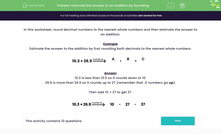### Comprehensive & curriculum aligned

In this worksheet, students will round decimal numbers to the nearest whole number in order to estimate the answer to an addition.Key stage:  KS 2

Curriculum topic:   Number: Fractions, Decimals and Percentages

Curriculum subtopic:   Solve Rounding Problems

Popular topics:   Subtraction worksheets, Addition worksheets, Rounding worksheets

Difficulty level:#### Worksheet Overview

In this worksheet, we are going to round decimal numbers to the nearest whole number and then estimate the answer to an addition.

How to round decimals to whole numbers

Look at the number directly to the right of the decimal point, that is the number in the tenths position.

If that number is less than 5, we round down so that we simply remove the numbers after the decimal point.

For example: 32.46 rounds down to 32 because the number in the tenths place is a 4.

159.08 rounds down to 159 because there is a zero in the tenths place.

If the number in the tenths place is 5 or more, we round up.

For example: 72.6 rounds up to 73 because the number in the tenths place is a 6.

197.57 rounds up to 198 because the number in the tenths place is a 5.

Let's use this knowledge to go one step further.

Estimate the answer to this addition by first rounding both decimals to the nearest whole number.

10.3 26.9A
+
 B
=
 C

10.3 has a 3 in the tenths position, so it rounds down to 10.

26.9 has a 9 in the tenths position, so it rounds up to 27.

Then add 10 + 27 to get 37.

10.3 26.910
+
 27
=
 37

Let's move on to some questions now.

### What is EdPlace?

We're your National Curriculum aligned online education content provider helping each child succeed in English, maths and science from year 1 to GCSE. With an EdPlace account you’ll be able to track and measure progress, helping each child achieve their best. We build confidence and attainment by personalising each child’s learning at a level that suits them.

Get started